MAXIMA AND MINIMA Analysis

• A function y=f(x) reaches a local MAXIMUM at a point xo when f(x)£f(xo) in the neighbourhood of x

• Similarly we can say that it reaches a local MINIMUM at a point xo when f(x)³f(xo) in the neighbourhood of x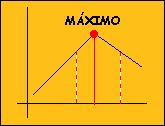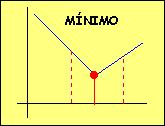Let's see what happens when we are working with derived functions.

 1.Local maxima and minima 2.Calculating maxima and minima 3.Applications

1. LOCAL MAXIMA AND MINIMA

A function y=f(x) reaches a local MAXIMUM at xo when f(x)£f(xo) in the neighbourhood of x

 Change the value of x on the window and check that, in this case, the function INCREASES then DECREASES. Note that the tangent is HORIZONTAL

Therefore: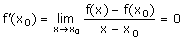Similarly y=f(x) reaches a Local MINIMUM at xo when f(x)³f(xo) in the neighbourhood of x

 Now the function first DECREASES then INCREASES. The tangent is also HORIZONTAL.

 If a function has a derivative and reaches a maximum or minimum at point xo , then f'(xo) = 0
• Although this condition is necessary it is not enough as a function could have a derivative of zero at a certain point but not have a maximum or minimum at this point.

Now look carefully at the curves of the function y=f(x),its derivative y=f'(x) and the second derivative y=f''(x) in the window.

 As you can see, f reaches a maximum when x=-1 and a minimum when x=1. Change the value of x in the window and look carefully at the values of f' and f'' at these points.
• What are the the maximum and minimum values?

• What is the relation between the sign of the second derivative and whether f reaches a maximum or minimum?

 If f'(xo) = 0 and f''(xo) <0, f reaches a maximum  at xo
 If f'(xo)=0 and f''(xo)>0, f reaches a minimum at xo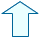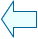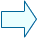María José García CebrianSpanish Ministry of Education. Year 2001